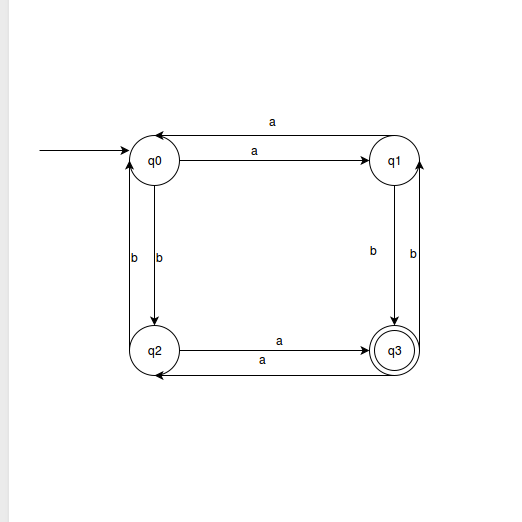# UGC-NET | UGC NET CS 2015 Jun – III | Question 21

The transition function for the language L = {w|na (w) and nb(w) are both odd} is given by:
δ (q0, a) = q1 ; δ (q0, b) = q2
δ (q1, a) = q0 ; δ (q1, b) = q3
δ (q2, a) = q3 ; δ (q2, b) = q0
δ (q3, a) = q2 ; δ (q3, b) = q1
The initial and final states of the automata are:
(A) q0 and q0respectively
(B) q0 and q1respectively
(C) q0 and q2respectively
(D) q0 and q3respectively